# Topological ring

(diff) ← Older revision | Latest revision (diff) | Newer revision → (diff)

A ringthat is a topological space, such that the mappings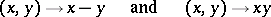are continuous. A topological ringis called separated if it is separated as a topological space (cf. Separation axiom). In this caseis a Hausdorff space. Any subringof a topological ring, and also the quotient ring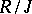by an ideal, is a topological ring. Ifis separated and the idealis closed, thenis a separated topological ring. The closure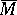of a subringinis also a topological ring. A direct product of topological rings is a topological ring in a natural way.

A homomorphism of topological rings is a ring homomorphism which is also a continuous mapping. If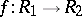is such a homomorphism, whereis moreover an epimorphism and an open mapping, then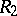is isomorphic as a topological ring to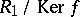. Banach algebras are an example of topological rings. An important type of topological ring is defined by the property that it has a fundamental system of neighbourhoods of zero consisting of some set of ideals. For example, to any ideal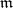in a commutative ringone can associate the-adic topology, in which the setsfor all natural numbersform a fundamental system of neighbourhoods of zero. This topology is separated if the conditionis satisfied.

For a topological ringone can define its completion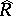, which is a complete topological ring, and a separated topological ringcan be imbedded as an everywhere-dense subset in, which is also separated in this case. The additive group of the ringcoincides with the completion of the additive group of, as an Abelian topological group.